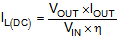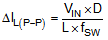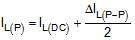SLVSFH6C January   2021  – December 2021

PRODUCTION DATA

1. Features
2. Applications
3. Description
4. Revision History
5. Pin Configuration and Functions
6. Specifications
7. Detailed Description
1. 7.1 Overview
2. 7.2 Functional Block Diagram
3. 7.3 Feature Description
4. 7.4 Device Functional Modes
8. Application and Implementation
1. 8.1 Application Information
2. 8.2 Typical Application – 3.6-V Output Boost Converter with Bypass
1. 8.2.1 Design Requirements
2. 8.2.2 Detailed Design Procedure
3. 8.2.3 Application Curves
4. 8.2.4 Typical Application – 3.3-V Output Boost Converter with Automatic Buck or Boost Function
9. Power Supply Recommendations
10. 10Layout
11. 11Device and Documentation Support
12. 12Mechanical, Packaging, and Orderable Information

• DSS|12
• DSS|12

#### 8.2.2.3 Inductor Selection

Because the selection of the inductor affects steady-state operation, transient behavior, and loop stability, the inductor is the most important component in power regulator design. There are three important inductor specifications: inductor value, saturation current, and DC resistance (DCR).

The TPS61094 is designed to work with 1-µH or 2.2-µH inductor values. Follow Equation 5 to Equation 7 to calculate the inductor peak current for the application. To calculate the current in the worst case, use the minimum input voltage, maximum output voltage, and maximum load current of the application. To have enough design margins, choose the inductor value with –30% tolerances and low power-conversion efficiency for the calculation.

In a boost regulator, the inductor DC current can be calculated by Equation 5.

Equation 5.where

• VOUT is the output voltage of the boost converter.
• IOUT is the output current of the boost converter.
• VIN is the input voltage of the boost converter.
• η is the power conversion efficiency, use 90% for most applications.

The inductor ripple current is calculated by Equation 6.

Equation 6.where

• D is the duty cycle, which can be calculated by Equation 2.
• L is the inductance value of the inductor.
• fSW is the switching frequency.
• VIN is the input voltage of the boost converter.

Therefore, the inductor peak current is calculated by Equation 7.

Equation 7.Normally, it is advisable to work with an inductor peak-to-peak current of less than 40% of the average inductor current for maximum output current. A smaller ripple from a larger-valued inductor reduces the magnetic hysteresis losses in the inductor and EMI, but in the same way, load transient response time is increased. The saturation current of the inductor must be higher than the calculated peak inductor current. Table 8-2 lists the recommended inductors for the TPS61094.

Table 8-2 Recommended Inductors for the TPS61094
PART NUMBER L (µH) DCR MAX (mΩ) SATURATION CURRENT (A) SIZE (LxWxH) VENDOR(1)
XGL4020-222ME 2.2 21.5 4.4 4.0 × 4.0 × 2.1 Coilcraft
VCHA042A-2R2MS6 2.2 23.0 4.5 4.3 x 4.3 x 2.1 Cyntec
744383560 22 2.2 35.0 6.2 4.1 x 4.1 x 2.1 Wurth Elecktronik
See the Third-Party Products disclaimer